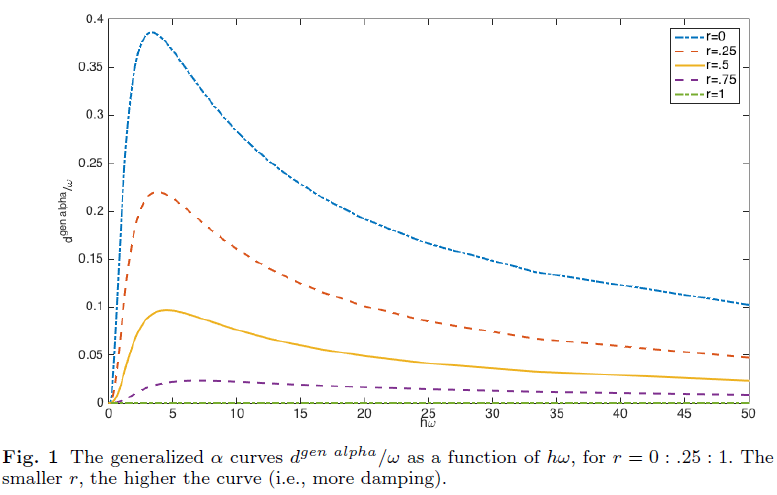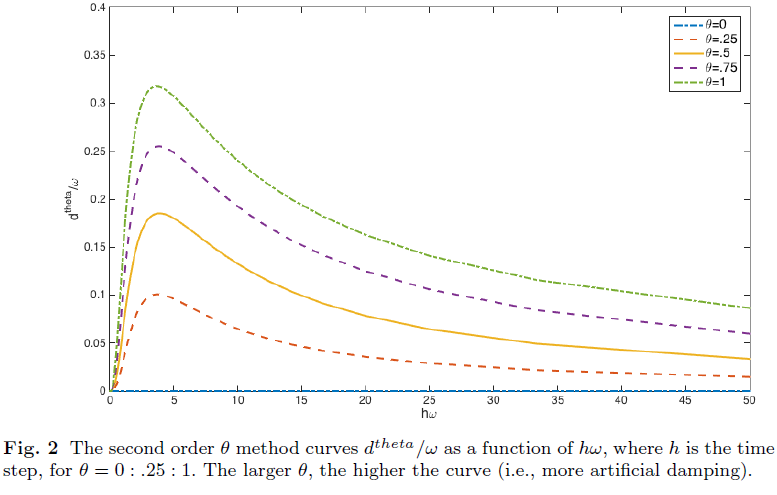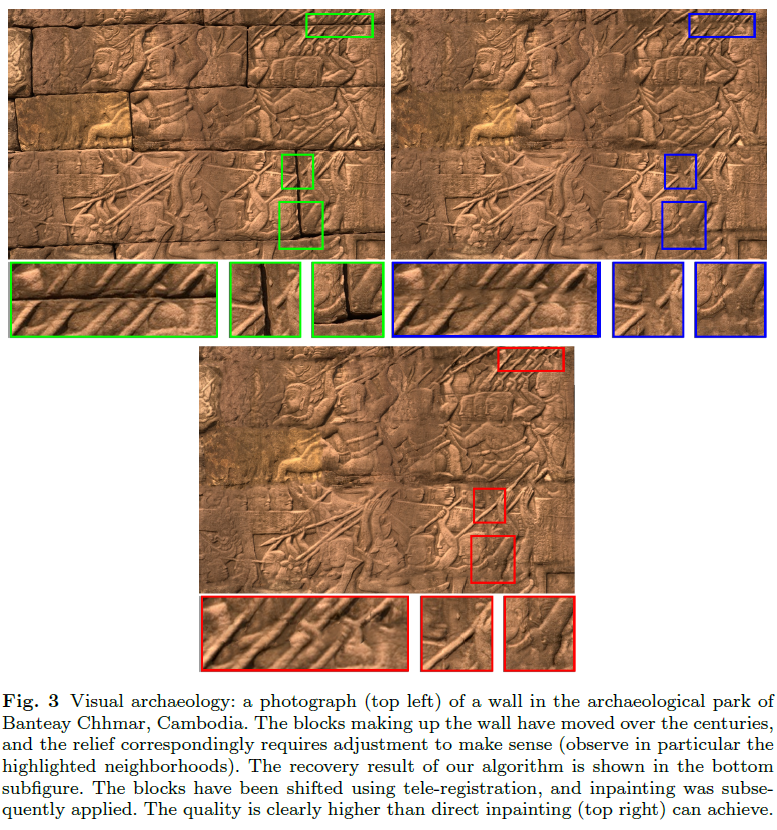### Numerical Analysis in Visual Computing What we can learn from each other

Abstract

Visual computing is a wide area that includes computer graphics and image processing, where the"eye-ball norm" rules.

This paper discusses two case studies involving numerical methods and analysis applied to this wide domain. The focus is on highlighting relative strengths in those intersection areas where there are many problems of interest both to numerical analysts and to researchers in the visual computing community.

The first involves motion simulation and calibration of soft objects such as cloth, plants and skin. The governing elastodynamics PDE system, discretized in space already at the variational level using co-rotated FEM, leads to a large, expensive to assemble, dynamical system in time, where the damped motion may mask highly oscillatory stiffness. Geometric integration and exponential time differencing ideas are making their way into visual computing research these days in search for more quantitative computations that are required for applications such as control and 3D-printing.

The other case study involves some image processing problems where there is a premium for local approaches that do not necessarily use underlying PDEs. The popular paradigm of solving a data fitting problem with a penalty term involving the gradient or higher derivatives of a function approximating the sought surface is employed in the visual community much less often than in thenumerical analysis community. We explain why. Concepts are demonstrated and discussed.Conclusions
We conclude with the following comments and observations:
- Incorporating more mathematically sound techniques into methods and algorithms for computer graphics and image processing can be a rather worthwhile cause.
- Significant practical advantages have been gained in visual computing using techniques involving physics-based simulation and data-driven model calibration.
- One may occasionally be able to use mathematical analysis to obtain solid justification of algorithms: both on when they work and on when they won't.
- Occasionally it is even possible to bridge the gap between qualitative and quantitative (which is our secret dream).
- However, at the same time, our advice to our younger colleagues is not to get swayed by sheer mathematical prowess.
- Watch out for situations where the gap between physics and physics-based appears to be too wide.
- Insisting on solving differential equations or satisfying mathematical topology theorems might lead to inferior algorithms for visual tasks: let us remember to keep our eyes on the ball, so to speak.

Bibtex
@ARTICLE{AH18,
title = {Numerical Analysis in Visual Computing: What we can learn from each other},
author = {Uri Ascher and Hui Huang},
journal = {Vietnam Journal of Mathematics},

volume = {46},
pages = {1–15},
year = {2018}
}